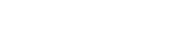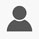Get 15%OFF On All Courses. Apply Code EDUSITY15
Price \$243.9
Enroll Now

#### Approx 20 Hours To Complete Master The Fundamental Of Math in English Language

Learn everything from the basics of math arithmetic, geometry, fractions, Algebra, trigonometry, and calculus then test your knowledge with practice questions. This course cover decimals, fraction, radicals, ratio, and proportion, trigonometry, arithmetic, and calculus.

#### Requirements

•Basic Understanding of calculus, factors and multiples

•Basic understanding of trigonometry

•Proper internet connection

•Basic understanding of scientific notation

#### FAQ

Q: What will I able to do upon completing the Mathematics course?

A: You will able to do calculus, trigonometry, and large amount of multiplication, addition, etc.

Q: What background knowledge is necessary?

A: Basic trigonometry and calculus knowledge necessary.

Q: You are able to solve which type of equation?

A: You will able to solve Trigonometry, calculus, fraction, Linear equation, decimal and fraction equation etc.

#### What you will learn

• Introduction

• Factors And Multiples

• Decimals

• Fractions

• Mixed Numbers

• Ratio And Proportion

• Exponents

• Scientific Notation

• Quick Guide• 0 Instructor Rating
• 0 Reviews
• 0 Students
• 5 Courses
• #### Sandhya Rajput

Skills :Academics,Accounting & Tax,Personal Well Being,SEO,IT And Tech

• Stars 5
• %0

• Stars 4
• %0

• Stars 3
• %0

• Stars 2
• %0

• Stars 1
• %0

## 0

Course Rating

Price \$243.9
Enroll Now
Get 15% OFF Use Code EDUSITY15

##### Includes
Do You have a Question

#### Course Features

• Lectures 11
• Quizzes 0
• Duration 20 hours
• Skill level 3
• Language English
• Assessments Yes

#### Skills You Will Acquire

• Mathematics
• Class 10th math